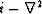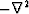# Factorization of cross spectra

Jon Claerbout, jon/res/xkolmog/

jon@sep.stanford.edu

A solved problem is the factorization of a positive real autospectrum into a minimum-phase wavelet and its adjoint. The most practical method is that of Kolmogoroff. Here I extend the Kolmogoroff method to cross-spectra.

This problem arises in the extrapolation of 3-D wavefields where we need to factor an operator like.We get a band matrix to solve. In principle, we factor it into lower and upper triangular band matrices which we then backsolve. Except at the ends, this is equivalent to a filter problem where the two backsubstitutions are polynomial divisions, one causal, the other anticausal. Althoughis an autocorrelation,is not, so we need two different minimum-phase filters whereas the Kolmogoroff method gives us the same one for both the causal and anticausal operations.